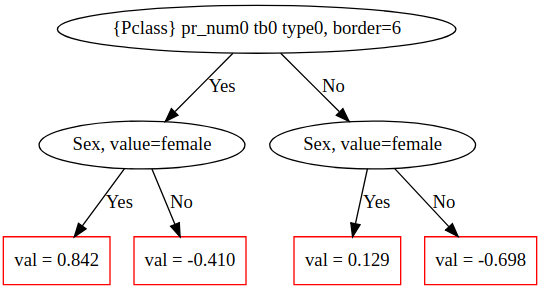plot_tree

Visualize the CatBoost decision trees.
Note. This method is suitable only for the set by default SymmetricTree tree growing policy (set in the grow_policy parameter).

graphviz

Method call format

plot_tree(tree_idx, pool=None)

Parameters

Parameter Possible types Description Default value
tree_idx int

The index of the tree from the model that should be visualized.

Required parameter
pool catboost.Pool

An optional parameter for models that contain only float features. Allows to pass a pool and label features with their external indexes from this pool. If the pool is not input, internal indexes are used.

For example, for a semicolon-separated pool with 2 features “f1;label;f2” the external feature indexes are 0 and 2, while the internal indexes are 0 and 1 respectively.

None

Required for models with one-hot encoded categorical feature

Parameter Possible types Description Default value
tree_idx int

The index of the tree from the model that should be visualized.

Required parameter
pool catboost.Pool

An optional parameter for models that contain only float features. Allows to pass a pool and label features with their external indexes from this pool. If the pool is not input, internal indexes are used.

For example, for a semicolon-separated pool with 2 features “f1;label;f2” the external feature indexes are 0 and 2, while the internal indexes are 0 and 1 respectively.

None

Required for models with one-hot encoded categorical feature

Type of return value

graphviz.dot.Digraph

Usage examples

import numpy as np
import catboost
from catboost import CatBoostClassifier, Pool

from catboost.datasets import titanic
titanic_df = titanic()

X = titanic_df.drop('Survived',axis=1)
y = titanic_df.Survived

is_cat = (X.dtypes != float)
for feature, feat_is_cat in is_cat.to_dict().items():
if feat_is_cat:
X[feature].fillna("NAN", inplace=True)

cat_features_index = np.where(is_cat)
pool = Pool(X, y, cat_features=cat_features_index, feature_names=list(X.columns))

model = CatBoostClassifier(
max_depth=2, verbose=False, max_ctr_complexity=1, iterations=2).fit(pool)

model.plot_tree(
tree_idx=0,
pool=pool
)

An example of a plotted tree:Tutorial

Refer to the Visualization of CatBoost decision trees tutorial for details.